# Transversality condition

A necessary condition for optimality in variational problems with variable end-points. The arbitrary constants on which the solution of the Euler equation depends are determined by means of the tranversality condition. The transversality condition is a necessary condition for the vanishing of the first variation of a functional.

For the simplest problem in variational calculus with variable end-points,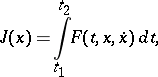in which the point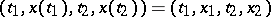is not fixed but can belong to a certain manifold, the transversality condition can be written in the form of the relation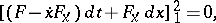(1)

which must be satisfied for any values of the tangent differentials,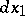,,of the boundary condition manifold.

If the left- and right-hand end-points of the extremal can be displaced along prescribed curves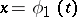and, then since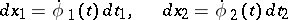and the variations ofand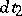are independent, (1) implies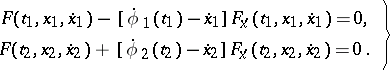(2)

If the equations of the curves along which the left- and right-hand end-points are displaced are given in implicit form,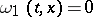and, then the transversality condition (1) can be written in the form(3)

If there are no constraints on one of the end-points, then at this end-point, by virtue of the independence of the respective tangent differentials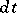and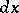, the transversality condition takes the form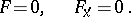(4)

The relations (2), (3), (4) are called transversality conditions.

Below, the transversality condition is given in the more general case of the variational problem for a conditional extremum. Consider the Bolza problem, i.e. the problem of minimizing a functional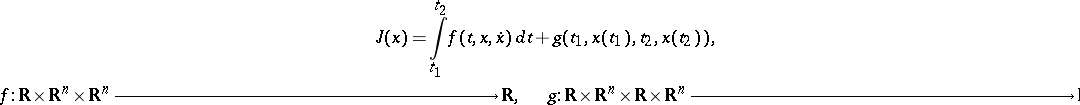(5)

in the presence of differential constraints of equality type,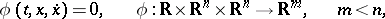(6)

and boundary conditions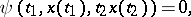(7)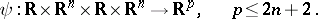Whenin this problem, the end-points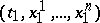andof the extremal are not fixed, but can be displaced along given hypersurfaces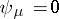,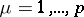.

In accordance with the transversality condition, there exist constants (Lagrange multipliers)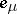,, as well as multipliersand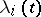,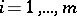, such that, in addition to the boundary conditions (7), the following relation holds at the end-points of the extremal:(8)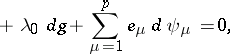for any choice of tangent differentials(9)

of the manifold defined by (7). In (8),denotes the expression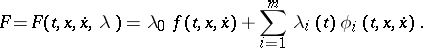(10)

In the majority of practical problems, the Lagrange multipliers are normalized by setting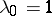(the valuecorresponds to an abnormal case, see ). The multipliers,, are determined together with the,, from the solution of the Euler system of differential equations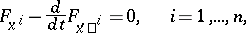(11)

andequations of the form (6):The general solution of this system ofsecond-order differential equations andfirst-order differential equations in theunknown functions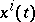,, and,, depends onarbitrary constants. In fact, if one sets(12)

then one obtains a system (11), (12) offirst-order differential equations andfinite relations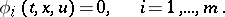(13)

Using (13), someof the functions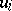can be expressed in terms of the others (under the hypothesis that the corresponding functional determinant does not vanish) and, on substituting these in (11), (12), one obtains a system of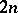first-order differential equations withunknown functions, the general solution of which depends onarbitrary constants. Along with the values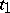and, this gives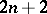arbitrary constants, determining the solution of the variational problem (5)–(7). One then obtains by means of the transversality condition the correct number of equations enabling one to determine these arbitrary constants.

In problems of optimal control and in the Pontryagin maximum principle, the necessary transversality condition is written similarly to (8), only instead of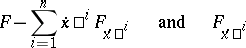one has to substitute in (8) the Hamiltonian, taken with the opposite sign, and the conjugate variables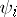.

The necessary transversality condition gives the missing boundary conditions for obtaining a closed boundary value problem to which the solution of the variational problem with variable end-points reduces.

How to Cite This Entry:
Transversality condition. Encyclopedia of Mathematics. URL: http://encyclopediaofmath.org/index.php?title=Transversality_condition&oldid=53948
This article was adapted from an original article by I.B. Vapnyarskii (originator), which appeared in Encyclopedia of Mathematics - ISBN 1402006098. See original article